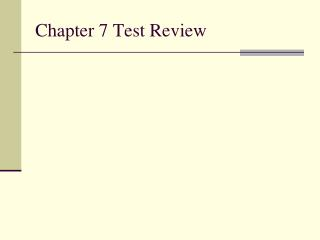# Chapter 7 Test Review - PowerPoint PPT PresentationDownload PresentationChapter 7 Test Review

Chapter 7 Test ReviewDownload Presentation## Chapter 7 Test Review

- - - - - - - - - - - - - - - - - - - - - - - - - - - E N D - - - - - - - - - - - - - - - - - - - - - - - - - - -
##### Presentation Transcript

1. Chapter 7 Test Review

2. 1. What is an ionic bond? Chemical bond resulting from the transfer of electrons from one atom to another.

3. 2. (Metals, nonmetals) tend to form positive ions and (metals, nonmetals) tend to form negative ions.

4. 3. Identify the metal and the nonmetal in the compound sodium chloride (NaCl). NaCl

5. 6. Write the empirical formula for the following ionic compounds: a. Al+3 and S-2: ___________________ b. Na+1 and F-1: ___________________ c. Na+1 and OH-1: _________________ d. NH4+1and CO3-2 : _______________

6. 7. Write the correct formula for each of the compounds. a. potassium iodide: ____________________ b. zinc chloride: ________________________ c. silver oxide: _________________________ d. sodium phosphide: ___________________

7. 8. Write the names for each of the following ionic compounds. a. LiI: _____________________________ b. AgI: ____________________________ c. NaN: ___________________________ d. MgO: ___________________________

8. 9. Write the molecular compounds using the prefix as the element’s subscript. a. diphosphorus pentaoxide: ____________ b. diarsenic trioxide: ___________________ c. dinitrogen monoxide: ________________ d. carbon dioxide: ____________________

9. 10. Write the names for each of the following molecular substances using prefixes. a. SiO2: _______________________ b. SF3 : _______________________

10. 11. Draw Lewis Dot Diagrams for the following elements. a. Nitrogen N b. Fluorine F

11. 12. Match the following Lewis Dash Structures to the type of bond it represents. a. N N 1. double bond  b. O – C - O 2. single bond H c. 3. triple bond H C H H

12. 13. Answer the following questions about magnesium hydroxide: Mg(OH)2. a. Name the elements in the compound.  b. How many total number of atoms are in the formula? c. Name the cation in the formula above. d. Is hydroxide (OH-1)a monatomic or polyatomic ion? How do you know? e. Is magnesium hydroxide formed from an ionic bond, covalent bond, or both? How do you know?